Next: Straight and Stepped Labyrinth Up: Fluid Section Types: Gases Previous: Bleed Tapping   Contents

### Preswirl Nozzle

A preswirl nozzle is a special kind of static orifice (Figure 88), used to impart a tangential velocity to gas before it enters a rotating device. That way, the loss due to the difference in circumferential velocity between the air entering the rotating device and the rotating device itself can be decreased. In the Figure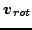is the rotational velocity of the orifice the preswirl nozzle is serving,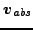is the absolute velocity of the air leaving the preswirl nozzle and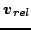is its velocity as seen by an observer rotating with the orifice (the so-called relative velocity). The velocity entering the calculation of the discharge coefficient of the rotating orifice is the tangential component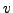of the velocity of the rotating device as seen by the air leaving the preswirl nozzle (which is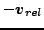). This velocity can be modified by a multiplicative factor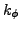.

The geometry of a preswirl nozzle can be quite complicated and the discharge coefficient should be ideally determined by experiments on the actual device. The basic equations are the same as for the orifice.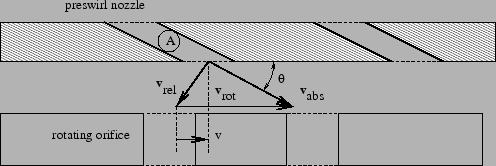The discharge coefficients provided by CalculiX are merely a rough estimate and are based on . For this purpose the preswirl nozzle must be described by the following constants (to be specified in that order on the line beneath the *FLUID SECTION, TYPE=PRESWIRL NOZZLE card):

• the cross section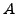.
•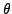(Figure 88) in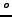.
•.
• the number of a curve (0 for the predefined curve).
• not used (internally: circumferential velocity at the outlet)

The angle at the exit of the nozzle is used to determine the circumferential velocity of the gas leaving the nozzle. This is stored for use in the (rotating) device following the nozzle. The curve number can be used to distinguish between several measured curves. Right now, only one curve is coded (number = 0 to select this curve). More specific curves can be implemented by the user, the appropriate routine to do so is cd_preswirlnozzle.f. Alternatively, the user can enter an own curve in the input deck listing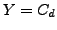versus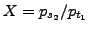. In that case the input reads

• the cross section.
•(Figure 88) in.
•.
• not used.
• not used (internally: circumferential velocity at the outlet).
• not used (internally: number of pairs).
•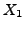.
•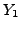.
•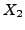.
•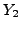.
• .. (maximum 16 entries per line; maximum 17 pairs in total)

Example files: moehring, vortex1, vortex2, vortex3.Next: Straight and Stepped Labyrinth Up: Fluid Section Types: Gases Previous: Bleed Tapping   Contents
guido dhondt 2018-12-15